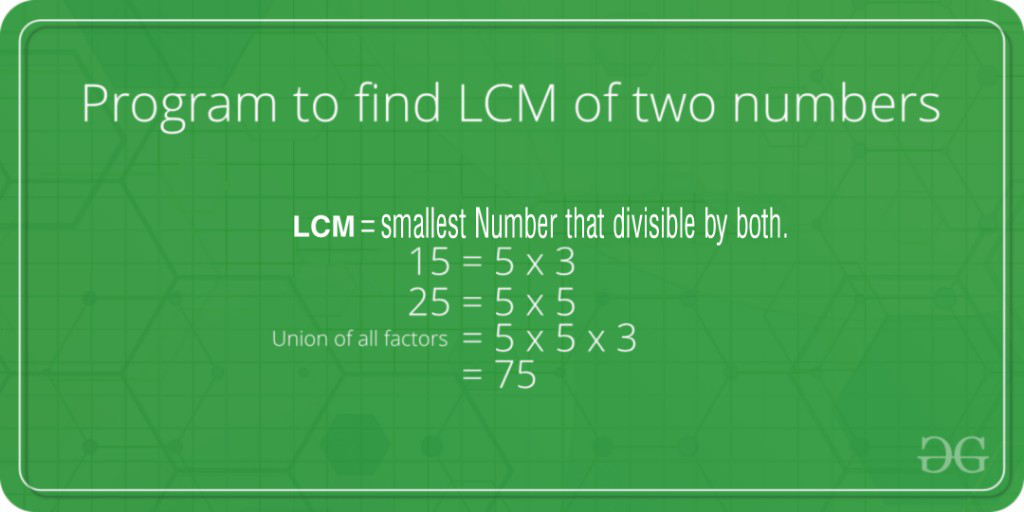# C Program To Find LCM of Two Numbers

• Last Updated : 19 Jul, 2022

LCM (Least Common Multiple) of two numbers is the smallest number which can be divided by both numbers.

For example, LCM of 15 and 20 is 60, and LCM of 5 and 7 is 35.A simple solution is to find all prime factors of both numbers, then find union of all factors present in both numbers. Finally, return the product of elements in union.

An efficient solution is based on the below formula for LCM of two numbers ‘a’ and ‘b’.

```   a x b = LCM(a, b) * GCD (a, b)

LCM(a, b) = (a x b) / GCD(a, b)```

We have discussed function to find GCD of two numbers. Using GCD, we can find LCM.

Below is the implementation of the above idea:

## C

 `// C program to find LCM ` `// of two numbers ` `#include `   `// Recursive function to return ` `// gcd of a and b ` `int` `gcd(``int` `a, ``int` `b) ` `{ ` `    ``if` `(a == 0)` `        ``return` `b; ` `    ``return` `gcd(b % a, a); ` `} `   `// Function to return LCM of ` `// two numbers ` `int` `lcm(``int` `a, ``int` `b) ` `{ ` `    ``return` `(a / gcd(a, b)) * b;` `} `   `// Driver code` `int` `main() ` `{ ` `    ``int` `a = 15, b = 20; ` `    ``printf``(``"LCM of %d and %d is %d "``, ` `            ``a, b, lcm(a, b)); ` `    ``return` `0; ` `} `

Output:

`LCM of 15 and 20 is 60`

Time Complexity: O(log(min(a,b))

Auxiliary Space: O(log(min(a,b))

Please refer complete article on Program to find LCM of two numbers for more details!

My Personal Notes arrow_drop_up
Recommended Articles
Page :